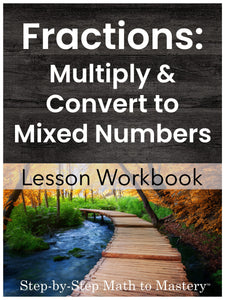# Fractions | Multiply Fractions and Convert to Mixed Numbers

• \$10.00
Unit price per
Shipping calculated at checkout.

## Multiplying Fractions by Whole Numbers (with Word Problems) | Convert Improper Fractions to Mixed Numbers

### 16 Step-by-Step Math Lessons | Special Education Math Intervention | Fourth Grade Math Level

This Multiplying Fractions and Converting Improper Fractions to Mixed Numbers lesson workbook includes an example IEP goal, 16 step-by-step math lessons, a review and assessment. This evidence-based math intervention is tied to fourth grade standards and is great for special education math goals and tier 2 small group math interventions (RTI).

These sequential lessons are easy to teach, with enough material for 3+ weeks of instruction!

These lessons introduce students to multiplying fractions.  Multiplying proper fractions can be introduced after students have learned to add and subtract fractions with like denominators.  A mix of multiplication, addition, and subtraction problems are included.  Students must learn to discriminate between the multiplication strategy (multiply across the top and bottom) and the addition/subtraction strategy (work only across the top).

An additional skill, converting improper fractions to mixed and whole numbers, is also taught in this workbook.  Students learn to recognize when a fraction is greater than 1 and then divide to find the equivalent mixed or whole number. Picture models are used in several lessons to promote understanding of concepts behind the steps.

Word problems involving multiplying a fraction and a whole number are included.  Plenty of space and visual structure is given for students to multiply and then divide if necessary to convert the answer to a mixed number.  Students are not asked to reduce fractions in this workbook.

16 Lessons
1 Review
1 Test

85 Pages

3+ Weeks of Instruction

## Students Will Be Able To:

• Multiply proper fractions
• Construct fractions equal to 1
• Find fractions greater than 1
• Rewrite fractions as mixed numbers
• Rewrite whole numbers as fractions
• Multiply a fraction by a whole number
• Multiply fractions by a whole number and convert answers to mixed or whole numbers
• Solve word problems

### Lessons Are Tied to These Fourth Grade Math Standards:

• Apply and extend previous understandings of multiplication to multiply a fraction by a whole number. (CCSS 4.NF.B.4)
• Solve word problems involving multiplication of a fraction by a whole number (CCSS 4.NF.B.4.c)

### Teacher Feedback:

❤️ “This resource is so perfect for teaching students with learning disabilities. It scaffolds the steps, sets a good pace, and provides repeated practice." -Sandra J K.

❤️ “This was a well-paced and put-together resource to help me in teaching my resource students! Thank you for the time and effort put into this!" -Nicole M.

❤️ “I love these materials. I have purchased most of the materials because it just makes the learning more structured for those who are struggling. They are able to feel successful before moving on!" -Tracey M.

❤️ Helped concepts make sense to a student with severe dyscalculia." -Stephanie M.

❤️ “This is a wonderful resource! It helps that the I Can statement appears at the top of each page! I use this in my self-contained classroom for students who are not yet able to perform on grade level. I researched for too long before finally finding this resource and I am NOT disappointed! Thank you for this!" -Jennifer M.

### Not sure where to start?

Watch a video overview of Step-by-Step Math to Mastery or read the transcript here.

### Step-by-Step Math to Mastery™ Resources:

Make math easier to understand.

• Help students over math hurdles with clear, sequential, scaffolded lessons.
• Prevent overwhelm. Build student confidence.

Make math easier to teach.

• Save hours of planning and piecing together materials.
• Paraeducators can deliver quality instruction independently. Open & teach.

### Reasons You'll Love Step-by-Step Math to Mastery™ Resources

• Boosts student confidence and progress
• Time-saving: print and teach
• Easy prep: only black ink is needed
• Easy to teach, paraeducator-friendly
• Example IEP goals and shorter term objectives
• Consistent & predictable format
• Lots of practice repetitions
• Scaffolded with structured workspaces
• Fewer problems on a page, white space, minimal visual clutter
• Tied to standards
• Explicit/Direct instruction
• Systematic & sequential
• Mastery approach--teaching one topic at a time, one strategy at a time
• Lessons have "I Can" statements, model problems, guided practice, & independent practice
• Each workbook can be used individually as a stand-alone intervention for that skill or they can be used together, taking students from the basics of number sense and addition in first grade all the way to dividing fractions and decimals in fifth grade.
• Can also be used with older students (middle & high school) to help fill the gaps in their learning.